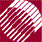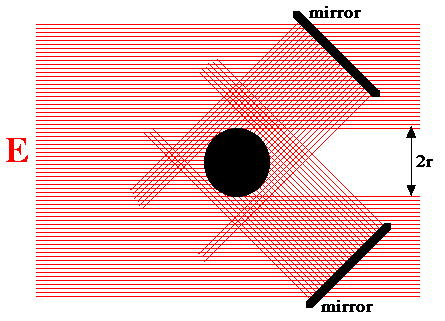ECE532 Biomedical Optics © 1998 Steven L. Jacques, Scott A. Prahl Oregon Graduate Institute

# F [W/cm2]

"At a given point in space, the radiant power incident on a small sphere divided by the cross-sectional area of that sphere."

(Often, fluence rate is denoted by the symbol φ. We choose the symbol F to avoid overuse of Greek symbols commonly used for angles. The use of F is not uncommmon.)

The Fluence Rate F [W/cm2] refers to the irradiance which is incident from all angles onto a small region of space. It finds common usage in describing the special case of total irradiance impinging on a target region of space within a turbid medium where light is being scattered toward the target from all directions.

Consider a broad uniform collimated beam of irradiance E striking a small black spherical target with radius r and cross-sectional area A = πr2 (see figure below). Place two mirrors behind the target off to the sides and at angles so that some of E which misses the target is redirected toward the target. Now the power that strikes the target and is absorbed by the target is:

Pabsorbed = 3EA

The fluence rate irradiating the target sphere is: F = Pabsorbed/A = 3E.The surface area of the sphere is not the factor that determines the amount of absorbed power. Rather, it is the cross-sectional area A which provides the "target size" for intercepting the primary irradiance and the secondary irradiance from the two mirrors.

In turbid media, the multiple scattering of photons causes light to be redirected toward a small target absorbing sphere from all directions. The situation is comparable to there being several mirrors redirecting the primary irradiance toward the target as secondary irradiance. The fluence rate is the total primary plus secondary irradiance impinging upon a sphere divided by the cross-sectional area of that sphere. In the limit of a very small sphere, the fluence rate F does not depend on the the size of the target sphere.